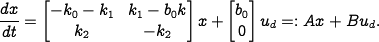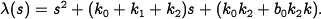Errata: Closed loop dynamics matrix is incorrect in Example 4.6

Location: page 107, line 12-23

The dynamics matrix of the closed loop system (on line 12) is incorrect. The displayed equation should readThis error propagates into the computation of the equilibrium pointin line 15, which should beFollowing this, the dynamics matrix in the next displayed equation (line 19) is again incorrect. The shifted, closed loop dynamics should beFinally, the characteristic equation on line 23 is incorrect and should instead be(Contributed by S. Han, 12 Oct 08)